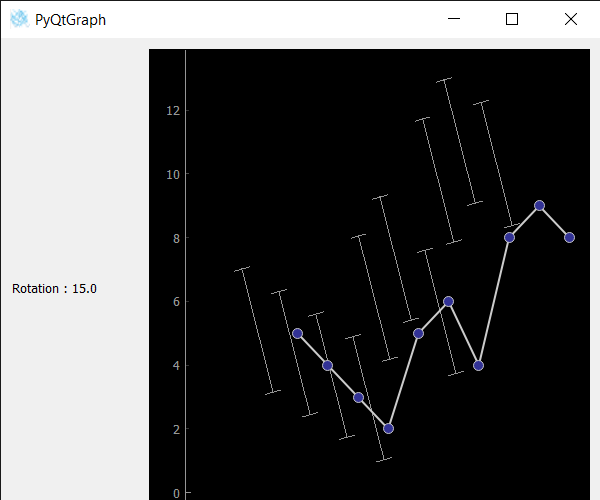GeeksforGeeks App
Open AppBrowser
Continue

# PyQtGraph – Getting Rotation Property of Error Bar Graph

In this article, we will see how we can get the rotation property of the bar graph in the PyQtGraph module. PyQtGraph is a graphics and user interface library for Python that provides functionality commonly required in designing and science applications. Its primary goals are to provide fast, interactive graphics for displaying data (plots, video, etc.). Error bars are graphical representations of the variability of data and used on graphs to indicate the error or uncertainty in a reported measurement. They give a general idea of how precise a measurement is, or conversely, how far from the reported value the true value might be. Rotation property rotates the data of the error bar graph taking origin as axis although this does not affect the actual line graph, only error graph data will be altered. It can be set with the help of setRotation method.
We can create a plot window and create an error bar graph on it with the help of commands given below.

```# creating a pyqtgraph plot window
plt = pg.plot()

# creating a error bar item object
error = pg.ErrorBarItem(x=x, y=y, top=top,
bottom=bottom, beam=0.5)```

In order to do this we use rotation method with the error bar item object
Syntax : error.rotation()
Argument : It takes no argument
Return : It returns float value

Below is the implementation.

## Python3

 `# importing Qt widgets``from` `PyQt5.QtWidgets ``import` `*` `# importing system``import` `sys` `# importing numpy as np``import` `numpy as np` `# importing pyqtgraph as pg``import` `pyqtgraph as pg``from` `PyQt5.QtGui ``import` `*``from` `PyQt5.QtCore ``import` `*` `from` `collections ``import` `namedtuple`  `class` `Window(QMainWindow):` `    ``def` `__init__(``self``):``        ``super``().__init__()` `        ``# setting title``        ``self``.setWindowTitle(``"PyQtGraph"``)` `        ``# setting geometry``        ``self``.setGeometry(``100``, ``100``, ``600``, ``500``)` `        ``# icon``        ``icon ``=` `QIcon(``"skin.png"``)` `        ``# setting icon to the window``        ``self``.setWindowIcon(icon)` `        ``# calling method``        ``self``.UiComponents()` `        ``# showing all the widgets``        ``self``.show()` `    ``# method for components``    ``def` `UiComponents(``self``):` `        ``# creating a widget object``        ``widget ``=` `QWidget()` `        ``# creating a label``        ``label ``=` `QLabel(``"Geeksforgeeks Error Bar plot"``)` `        ``# setting minimum width``        ``label.setMinimumWidth(``130``)` `        ``# making label do word wrap``        ``label.setWordWrap(``True``)` `        ``# setting configuration options``        ``pg.setConfigOptions(antialias``=``True``)` `        ``# creating x-axis values``        ``x ``=` `np.array([``1``, ``2``, ``3``, ``4``, ``5``, ``6``, ``7``, ``8``, ``9``, ``10``])` `        ``# creating y-axis values``        ``y ``=` `np.array([``5``, ``4``, ``3``, ``2``, ``5``, ``6``, ``4``, ``8``, ``9``, ``8``])` `        ``# creating upper bound values``        ``top ``=` `np.array([``2``, ``2``, ``2``, ``2``, ``2``, ``2``, ``2``, ``2``, ``2``, ``2``])` `        ``# creating lower bound values``        ``bottom ``=` `np.array([``2``, ``2``, ``2``, ``2``, ``2``, ``2``, ``2``, ``2``, ``2``, ``2``])` `        ``# creating a plot window``        ``plt ``=` `pg.plot()` `        ``# creating a error bar item``        ``error ``=` `pg.ErrorBarItem(beam``=``0.5``)` `        ``# setting data to error bar item``        ``error.setData(x``=``x, y``=``y, top``=``top, bottom``=``bottom)` `        ``# adding error bar item to the plot window``        ``plt.addItem(error)` `        ``# plotting the data on plot window``        ``plt.plot(x, y, symbol``=``'o'``, pen``=``{``'color'``: ``0.8``, ``'width'``: ``2``})` `        ``# Creating a grid layout``        ``layout ``=` `QGridLayout()` `        ``# minimum width value of the label``        ``label.setMinimumWidth(``130``)` `        ``# setting this layout to the widget``        ``widget.setLayout(layout)` `        ``# adding label in the layout``        ``layout.addWidget(label, ``1``, ``0``)` `        ``# plot window goes on right side, spanning 3 rows``        ``layout.addWidget(plt, ``0``, ``1``, ``3``, ``1``)` `        ``# setting this widget as central widget of the main window``        ``self``.setCentralWidget(widget)` `        ``# setting rotation of error bar item``        ``error.setRotation(``15``)` `        ``# getting rotation value``        ``value ``=` `error.rotation()` `        ``# setting text to the label``        ``label.setText(``"Rotation : "` `+` `str``(value))`  `# create pyqt5 app``App ``=` `QApplication(sys.argv)` `# create the instance of our Window``window ``=` `Window()` `# start the app``sys.exit(App.``exec``())`

Output:My Personal Notes arrow_drop_up